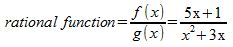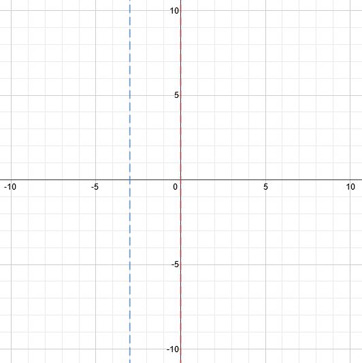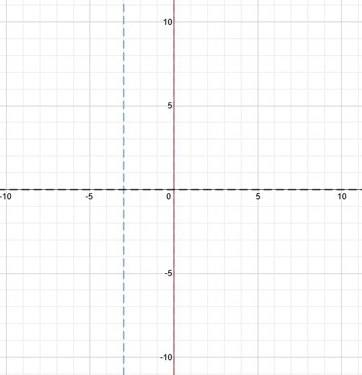# Graphing Rational Functions That Have Polynomials of Various Degrees: Steps & Examples

An error occurred trying to load this video.

Try refreshing the page, or contact customer support.

Coming up next: Analyzing the Graph of a Rational Function: Asymptotes, Domain, and Range

### You're on a roll. Keep up the good work!

Replay
Your next lesson will play in 10 seconds
• 0:49 What You Need
• 1:34 Finding Asymptotes
• 5:26 Finding Points
• 8:01 Lesson Summary

Want to watch this again later?

Timeline
Autoplay
Autoplay
Speed

#### Recommended Lessons and Courses for You

Lesson Transcript
Instructor: Yuanxin (Amy) Yang Alcocer

Amy has a master's degree in secondary education and has taught math at a public charter high school.

Graphing rational functions is not as hard or as scary as it sounds. Sure, the functions may be big, but watch this video lesson and you will see that graphing these functions can actually be easy.

## Rational Functions

Rational functions can be described as fractions made up of polynomial functions. A polynomial is an expression made up of variables with their coefficients separated by either addition or subtraction. If we had two polynomial functions that we have labeled f(x) and g(x), then our rational function can be written as f(x) / g(x). As an example, if my f(x) equaled 5x + 1 and my g(x) equaled x^2 + 3x, then my rational function will be (5x + 1) / (x^2 + 3x).It looks scary, doesn't it? Don't worry, though, graphing these rational functions isn't all that bad.

## What You Need to Graph

There are only a few things that you need to find to graph them, which include the vertical asymptotes, the horizontal asymptotes and the points to plot. A vertical asymptote is an x value that makes our function invalid. In other words, it is the x value that produces an error in our function when we try to evaluate our function at that value. Our function and graph will never be this x value.

A horizontal asymptote is the y value that the graph approaches as the x values get very large and very small. In other words, the horizontal asymptote is the value that you see the graph approaching to the far left of the graph and the far right of the graph.

## Finding the Asymptotes

To find the vertical asymptotes of our rational function, we simply set our denominator equal to 0 and solve. Why do we do this? If our vertical asymptote is the x value that our graph cannot be, then our best way to find out these values is to set the denominator equal to 0 because it is at those values that the function is not valid. We cannot divide by 0, so by finding the x values when our denominator equals 0, we find those x values the function cannot be.

Let's use the rational function we began with and find its vertical asymptotes. We set the denominator equal to 0:
x^2 + 3x = 0

We solve for x by using algebra. Our denominator is a quadratic, so we will use factoring to solve:
x(x + 3) = 0

We set both factors equal to 0 and solve for each.
x = 0 and x + 3 = 0

We have two x values here, actually. We have x = 0, and we have x = -3. So these are our two vertical asymptotes.

We can begin graphing our data by drawing dashed lines to represent our vertical asymptotes at x = 0 and x = -3.Next, we need to find our horizontal asymptotes. To do this, we compare the first term of our numerator to the first term of our denominator. What we are looking for is the exponent of this term. There are three scenarios that we will look for in helping us find our horizontal asymptote.

The first scenario is when the exponent of the numerator is higher than the exponent of the denominator. When this happens, the y values of the graph will go towards either positive infinity or negative infinity as the x value gets very large and very small. Looking at our rational function, we see that this is not our case, so let's look at the second case.

The second case is when the exponent of the first term of the numerator is the same as the exponent of the first term in the denominator. When this is the case, we find the horizontal asymptote by dividing the coefficient of the first term of the numerator by the coefficient of the first term of the denominator. For example, if our rational function is (5x + 1) / (3x + 2), then our horizontal asymptote is y = 5/3. Is this our case? Looking at our function again, we see that this is also not our case. So we move on to look at the third scenario.

The third scenario is when the exponent of the first term in the numerator is smaller than the exponent of the first term in the denominator. When this happens, our horizontal asymptote is the line y = 0. Looking at our function, we can see that yes, this is our case. So that means we have a horizontal asymptote at y = 0. We can mark this with a dashed line as well. We recall that while it is never okay for our graph to cross a vertical asymptote, it is perfectly okay for the graph to cross a horizontal asymptote.How can you remember these? Picture a mean fraction with big teeth that gets angry when you touch its ticklish spots, or its vertical asymptotes. When is the fraction ticklish? It's ticklish when the denominator is 0. Then picture the fraction monster running towards a piece of pie. This is the horizontal asymptote, or the behavior when the fraction monster is getting further and further away. The horizontal asymptote is determined by the first term of the numerator and denominator or what the monster is made of.

To unlock this lesson you must be a Study.com Member.

### Register to view this lesson

Are you a student or a teacher?

### Unlock Your Education

#### See for yourself why 30 million people use Study.com

##### Become a Study.com member and start learning now.
Back
What teachers are saying about Study.com

### Earning College Credit

Did you know… We have over 160 college courses that prepare you to earn credit by exam that is accepted by over 1,500 colleges and universities. You can test out of the first two years of college and save thousands off your degree. Anyone can earn credit-by-exam regardless of age or education level.